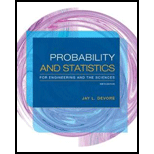Probability and Statistics for Engineering and the Sciences
9th Edition
ISBN: 9781305251809
Author: Jay L. Devore
Publisher: Cengage LearningProbability and Statistics for Engineering and the Sciences
Joint Probability Distributions And Random Samples. 91SE

# Prove that X 1 + X 2 has a chi-squared distribution with parameter ν 1 + ν 2 .

QuestionChapter 5, Problem 91SE

a.

To determine

## Prove that X1+X2 has a chi-squared distribution with parameter ν1+ν2.

a.

Expert Solution

### Explanation of Solution

Given info:

Reference- Example 5.22: The random variables X1 and X2 are independently distributed with chi-squared distribution. The parameter of X1 is ν1 and that of X2 is ν2.

Calculation:

The probability density function (pdf) of a random variable X having a chi-squared distribution with parameter ν is given as:

fX(x)={12ν2Γ(ν2)xν21ex2, x00,                         otherwise.

Denote To=X1+X2. Thus, using the above pdf and the technique in Example 5.22, the cdf of To is obtained as follows:

FTo(t)=P(X1+X2t)=0t0tx1[12ν12Γ(ν12)x1ν121ex12][12ν22Γ(ν22)x2ν221ex22]dx2dx1 (as x1+x2=t)=1(2ν12Γ(ν12))(2ν22Γ(ν22))0t0tx1x1ν121x2ν221ex1+x22dx2dx1=1(2ν12Γ(ν12))(2ν22Γ(ν22))0t0tx1x1ν121x2ν221et2dx2dx1

Make the transformations:

x1=tp and x2=t(1p), so that x1+x2=t is true and 0p1.

The Jacobian determinant of the transformation is:

|J(t,p)|=|x1t x1px2t x2p|=|p             t(1p) t|=|tpt+tp|=t.

In that case, the above integral becomes:

FTo(t)=1(2ν12Γ(ν12))(2ν22Γ(ν22))0t01(tp)ν121(t(1p))ν221et2|J(t,p)|dpdt=et22ν1+ν22Γ(ν1+ν22)β(ν12,ν22)0ttν121+ν22101pν121(1p)ν221tdpdt=et22ν1+ν22Γ(ν1+ν22)0ttν12+ν221[1β(ν12,ν22)01pν121(1p)ν221dp]dt.

Now, consider the quantity:

1β(ν12,ν22)01pν121(1p)ν221dp.

This is the integration of the probability density function (pdf) of the beta distribution (first kind) taken over the whole range (0,1). Hence,

1β(ν12,ν22)01pν121(1p)ν221dp=1.

Thus, the cdf reduces to:

FTo(t)=et22ν1+ν22Γ(ν1+ν22)0ttν12+ν221[1β(ν12,ν22)01pν121(1p)ν221dp]dt=et22ν1+ν22Γ(ν1+ν22)0ttν12+ν221dtFTo(t)=0tet22ν1+ν22Γ(ν1+ν22)tν12+ν221dt_.

Compare this with the pdf of the chi-squared distribution. It is clearly observed that the quantity within the integral, et22ν1+ν22Γ(ν1+ν22)tν12+ν221, is the pdf of a chi-squared distribution where the degrees of freedom is ν=ν1+ν22.

It is known that the cdf of a distribution is unique. Thus, using the uniqueness property of the cdf of a random variable, it is proved that X1+X2 has a chi-squared distribution with parameter ν1+ν2_.

b.

To determine

### Find the distribution of Z12+Z22+...+Zn2.

b.

Expert Solution

The distribution of Z12+Z22+...+Zn2 is a chi-squared distribution with parameter ν=n_.

### Explanation of Solution

Given info:

Reference- Exercise 71: The random variables Z1,Z2,...,Zn are independently distributed standard normal variables.

Calculation:

The result in Exercise 71 shows that, for a standard normal random variable, Z, the variable Z2 has a chi-squared distribution with ν=1.

The result in part a shows that the addition of two variables having chi-squared distributions with parameters ν1 and ν2 produces another variable, which also a chi-squared distribution with parameter ν=ν1+ν2. This result can be generalized for the addition of n such variables with chi-squared distributions.

For the random variables Z1,Z2,...,Zn, each Zi2 has a chi-squared distribution with νi=1, for i=1,2,...,n.

Thus, by generalization of the result in part a and the result in Exercise 71, the random variable Z12+Z22+...+Zn2 will have a chi-squared distribution. The parameter of this distribution is:

1+1+...+1 (upto n terms)=i=1n(1)=n.

Hence, the distribution of Z12+Z22+...+Zn2 is a chi-squared distribution with parameter ν=n_.

c.

To determine

### Find the distribution of the sum Y=∑i=1n(Xi−μσ)2.

c.

Expert Solution

The distribution of the sum Y=i=1n(Xiμσ)2 is chi-squared distribution with parameter ν=n_.

### Explanation of Solution

Given info:

The random variables X1,X2,...,Xn form a random sample taken from a normal distribution with mean μ and variance σ2.

Calculation:

For a normally distributed random variable, X, with mean μ and variance σ2, the standard normal variable, Z, obtained is:

Z=Xμσ.

Thus, for the normally distributed random variables Xi each having n μ and variance σ2, the corresponding standard normal variable is

Zi=Xiμσ, for i=1,2,...,n.

Now,

Y=i=1n(Xiμσ)2=i=1nZi2=Z12+Z22+...+Zn2.

Thus, Y is the sum of the squares of n standard normal variables.

The result in part b shows that the distribution of Z12+Z22+...+Zn2 is a chi-squared distribution with parameter ν=n.

Hence, the distribution of the sum Y=i=1n(Xiμσ)2 is chi-squared distribution with parameter ν=n_.

### Want to see more full solutions like this?

Subscribe now to access step-by-step solutions to millions of textbook problems written by subject matter experts!Probability and Statistics for Engineering and the Sciences
9th Edition
ISBN: 9781305251809
Author: Jay L. Devore
Publisher: Cengage LearningProbability and Statistics for Engineering and the Sciences
Joint Probability Distributions And Random Samples. 91SEEstimationMore videos on
Point Estimation, Limit Theore...Want to see more full solutions like this?
Subscribe now to access step-by-step solutions to millions of textbook problems written by subject matter experts.
Knowledge BoosterNeed a deep-dive on the concept behind this application? Watch these videos for an in-depth look
Statistics 4.1 Point Estimators
Dr. Jack L. Jackson II
Statistics 101: Point Estimators
Brandon Foltz
Central limit theorem
365 Data Science
Point Estimate Definition & Example
Prof. Essa
Point Estimation
Vamsidhar Ambatipudi
Recommended textbooks for you
•MATLAB: An Introduction with Applications
Statistics
ISBN:9781119256830
Author:Amos Gilat
Publisher:John Wiley & Sons IncProbability and Statistics for Engineering and th...
Statistics
ISBN:9781305251809
Author:Jay L. Devore
Publisher:Cengage LearningStatistics for The Behavioral Sciences (MindTap C...
Statistics
ISBN:9781305504912
Author:Frederick J Gravetter, Larry B. Wallnau
Publisher:Cengage Learning
•Elementary Statistics: Picturing the World (7th E...
Statistics
ISBN:9780134683416
Author:Ron Larson, Betsy Farber
Publisher:PEARSONThe Basic Practice of Statistics
Statistics
ISBN:9781319042578
Author:David S. Moore, William I. Notz, Michael A. Fligner
Publisher:W. H. FreemanIntroduction to the Practice of Statistics
Statistics
ISBN:9781319013387
Author:David S. Moore, George P. McCabe, Bruce A. Craig
Publisher:W. H. Freeman
•MATLAB: An Introduction with Applications
Statistics
ISBN:9781119256830
Author:Amos Gilat
Publisher:John Wiley & Sons IncProbability and Statistics for Engineering and th...
Statistics
ISBN:9781305251809
Author:Jay L. Devore
Publisher:Cengage LearningStatistics for The Behavioral Sciences (MindTap C...
Statistics
ISBN:9781305504912
Author:Frederick J Gravetter, Larry B. Wallnau
Publisher:Cengage LearningElementary Statistics: Picturing the World (7th E...
Statistics
ISBN:9780134683416
Author:Ron Larson, Betsy Farber
Publisher:PEARSONThe Basic Practice of Statistics
Statistics
ISBN:9781319042578
Author:David S. Moore, William I. Notz, Michael A. Fligner
Publisher:W. H. FreemanIntroduction to the Practice of Statistics
Statistics
ISBN:9781319013387
Author:David S. Moore, George P. McCabe, Bruce A. Craig
Publisher:W. H. Freeman